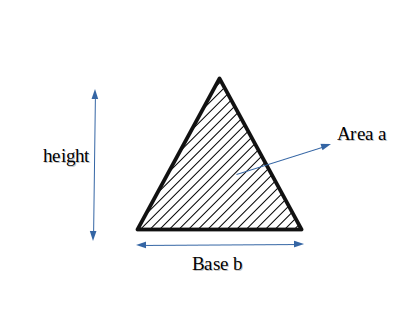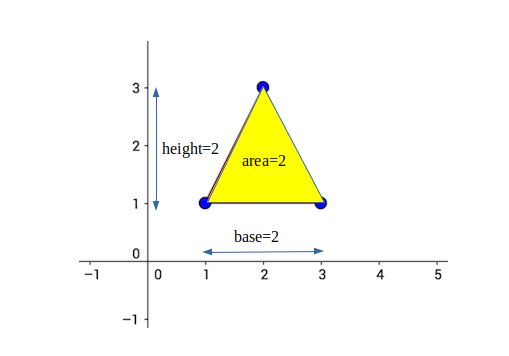Open In App
Related Articles

# Minimum height of a triangle with given base and area

Given two integers a and b, find the smallest possible height such that a triangle of at least area “a” and base “b” can be formed.Examples:

```Input : a = 2, b = 2
Output : Minimum height of triangle is 2

Explanation: ``````Input : a = 8, b = 4
Output : Minimum height of triangle is 4```

Minimum height of Triangle with base “b” and area “a” can be evaluated by having the knowledge of the relationship between the three.

The relation between area, base and
height is:
area = (1/2) * base * height
So height can be calculated as :
height = (2 * area)/ base
Minimum height is the ceil of the
height obtained using above formula.

Example:

## C++

 `#include ``using` `namespace` `std;``// function to calculate minimum height of``// triangle``int` `minHeight(``int` `base, ``int` `area)``{``    ``return` `ceil``((``float``)(2 * area) / base);``}` `int` `main()``{``    ``int` `base = 4, area = 8;``    ``cout << ``"Minimum height is "` `<< minHeight(base, area)``         ``<< endl;` `    ``return` `0;``}`

## Java

 `// Java code Minimum height of a``// triangle with given base and area` `class` `GFG {``    ` `    ``// function to calculate minimum height of``    ``// triangle``    ``static` `double` `minHeight(``double` `base, ``double` `area)``    ``{``        ``double` `d = (``2` `* area) / base;``        ``return` `Math.ceil(d);``    ``}``    ` `    ``// Driver code``    ``public` `static` `void` `main (String[] args)``    ``{``        ``double` `base = ``4``, area = ``8``;``        ``System.out.println(``"Minimum height is "``+``                            ``minHeight(base, area));``    ``}``}``// This code is contributed by Anant Agarwal.`

## Python

 `# Python Program to find minimum height of triangle``import` `math` `def` `minHeight(area,base):``        ``return` `math.ceil((``2``*``area)``/``base)` `# Driver code``area ``=` `8``base ``=` `4``height ``=` `minHeight(area, base)``print``(``"Minimum height is %d"` `%` `(height))`

## C#

 `// C# program to find minimum height of``// a triangle with given base and area``using` `System;` `public` `class` `GFG {` `    ``// function to calculate minimum``    ``// height of triangle``    ``static` `int` `minHeight(``int` `b_ase, ``int` `area)``    ``{``        ``return` `(``int``)Math.Round((``float``)(2 * area) / b_ase);``    ``}``    ` `    ``// Driver function``    ``static` `public` `void` `Main()``    ``{``        ``int` `b_ase = 4, area = 8;``        ``Console.WriteLine(``"Minimum height is "``                        ``+ minHeight(b_ase, area));``    ``}``}` `// This code is contributed by vt_m.`

## PHP

 ``

## Javascript

 ``

Output :

`Minimum height is 4`

Time complexity: O(1)
Auxiliary Space: O(1)

#### Approach#2: Using Heron’s formula

Heron’s formula is an alternative way to calculate the area of a triangle. Given the lengths of all three sides of a triangle (a, b, and c), the area can be calculated using the following formula: Area = ?(s(s-a)(s-b)(s-c)) where s is the semi perimeter of the triangle, which is half the perimeter: s = (a + b + c) / 2 Using this formula, we can rearrange it to solve for the height of the triangle in terms of the base and area: Height = (2 * Area) / Base

Algorithm:

1. Calculate the semiperimeter s using s = (a + b + c) / 2
2. Calculate the area of the triangle using Heron’s formula
3. Calculate the height of the triangle using Height = (2 * Area) / Base
4. Return the height as the output

## C++

 `#include ``#include ` `using` `namespace` `std;` `double` `minimumHeight(``double` `base, ``double` `area)``{``    ``double` `s = base / 2;``  ` `    ``// Calculate the area of the triangle using Heron's``    ``// formula``    ``double` `area_tri = (4 * ``pow``(area, 2)) / ``pow``(base, 2);` `    ``// Calculate the height of the triangle using Height =``    ``// (2 * Area) / Base``    ``double` `height = (2 * area) / base;``    ``return` `height;``}` `int` `main()``{``    ``double` `area = 8;``    ``double` `base = 4;``    ``cout << minimumHeight(base, area);``    ``return` `0;``}`

## Java

 `public` `class` `MinimumHeight {``  ``public` `static` `double` `minimumHeight(``double` `base, ``double` `area) {``    ``double` `s = base / ``2``;``    ``// Calculate the area of the triangle using Heron's formula``    ``double` `area_tri = (``4` `* Math.pow(area, ``2``)) / Math.pow(base, ``2``);` `    ``// Calculate the height of the triangle using Height = (2 * Area) / Base``    ``double` `height = (``2` `* area) / base;``    ``return` `height;``  ``}` `  ``public` `static` `void` `main(String[] args) {``    ``double` `area = ``8``;``    ``double` `base = ``4``;``    ``System.out.println(minimumHeight(base, area));``  ``}``}`

## Python3

 `def` `minimum_height(base, area):``  ``s ``=` `base ``/` `2``  ``# Calculate the area of the triangle using Heron's formula``  ``area_tri ``=` `(``4` `*` `area``*``*``2``) ``/` `(base``*``*``2``)` `  ``# Calculate the height of the triangle using Height = (2 * Area) / Base``  ``height ``=` `(``2` `*` `area) ``/` `base``  ``return` `height``area ``=` `8``base ``=` `4``print``(minimum_height(base, area))`

## Javascript

 `function` `minimumHeight(base, area) {``  ``let s = base / 2;``  ``// Calculate the area of the triangle using Heron's formula``  ``let areaTri = (4 * Math.pow(area, 2)) / Math.pow(base, 2);` `  ``// Calculate the height of the triangle using Height = (2 * Area) / Base``  ``let height = (2 * area) / base;``  ``return` `height;``}` `let area = 8;``let base = 4;``console.log(minimumHeight(base, area));`

Output

`4.0`

Time complexity: O(1)
Auxiliary Space: O(1)